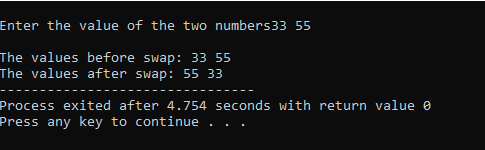# Pointer To Function In C Programming

As we have discussed earlier, a pointer is a variable which holds the address of another variable.

Here in a pointer to function, the address of the variable is passed to a function where the address is caught and used for its operation. The operation executed in that function is done only with the help of the pointer holding the address.

Let us see an example to get the things cleared:

### A program to swap the values of two variables using a pointer.

```#include<stdio.h>
int main()
{
int a,b;
printf("\nEnter the value of the two numbers");
scanf("%d%d",&a,&b);
printf("\nThe values before swap: %d %d",a,b);
swap(&a,&b);
printf("\nThe values after swap: %d %d",a,b);
return 0;
}
void swap(int *a, int *b)
{
int t;
t=*a;
*a=*b;
*b=t;
}```

In the above program the address values of variable a and b which holds the actual values are passed in the swap function, where the address values are swapped which ultimately results in the interchange of the actual values and then the variables are printed to get the result of the final operation.

Output:Hence, we come to the short and simple topic of pointer to function. Hope you like it and have cleared all your doubts.